# What is the correct format to export dates with html5 to excel?

These are my dates in datatables (dd/mm/yyyy).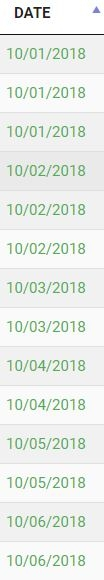Then I export these dates with the excel button.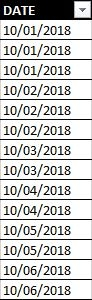As you can see the dates are in the left side and excel treats them as a general format.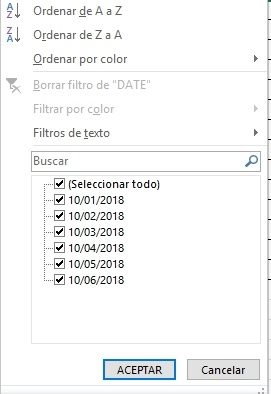Then if I double click each row excel treats theam as date format (right side of the column)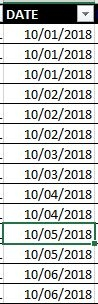and now I can use the date filters correctly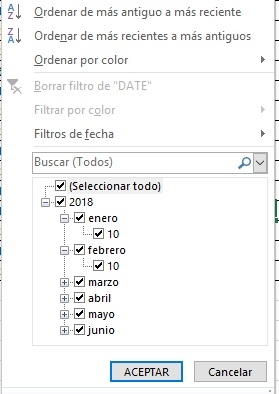I tried dd/mm/yyyy and yyyy-mm-dd formats and it does not work I always need to double click on each row.
The only thing I need is not to use the double click again each row.

«1

• Posts: 14,439Questions: 1Answers: 2,459

Hi @jfabian ,

These two threads here and here should help, it looks like people got it working in the past,

Cheers,

Colin

• Hi @colin neither of the two worked. I don't mind using ISO8601 dates
but it does not automatically detect them. It seems that @ajhulsebos or @mrmccrac are the closest but I can't get it to work.

• Posts: 14,439Questions: 1Answers: 2,459

Hi @jfabian ,

Would you be able to link to the page with the problem, or create a test case that reproduces the problem? That would help to understand the issue,

Cheers,

Colin

• @colin @allan I find the answer !!! (after a week).

First we have this line.

``````<cellXfs count="67">
``````

We change it to this

``````<cellXfs count="68">
``````

Then we have this second line.

``````   '<xf numFmtId="2" fontId="0" fillId="0" borderId="0" applyFont="1" applyFill="1" applyBorder="1" xfId="0" applyNumberFormat="1"/>'+
'</cellXfs>'+
``````

We add the line beetween. The number 14 is really important because is the date format.

``````  '<xf numFmtId="2" fontId="0" fillId="0" borderId="0" applyFont="1" applyFill="1" applyBorder="1" xfId="0" applyNumberFormat="1"/>'+
'<xf numFmtId="14" fontId="0" fillId="0" borderId="0" applyFont="1" applyFill="1" applyBorder="1" xfId="0" applyNumberFormat="1"/>'+
'</cellXfs>'+
``````

It also needs to change _excelSpecials.

``````var _excelSpecials = [
{ match: /^\-?\d+\.\d%\$/,       style: 60, fmt: function (d) { return d/100; } }, // Precent with d.p.
{ match: /^\-?\d+\.?\d*%\$/,     style: 56, fmt: function (d) { return d/100; } }, // Percent
{ match: /^\-?\\$[\d,]+.?\d*\$/,  style: 57 }, // Dollars
{ match: /^\-?£[\d,]+.?\d*\$/,   style: 58 }, // Pounds
{ match: /^\-?€[\d,]+.?\d*\$/,   style: 59 }, // Euros
{ match: /^\-?\d+\$/,            style: 65 }, // Numbers without thousand separators
{ match: /^\-?\d+\.\d{2}\$/,     style: 66 }, // Numbers 2 d.p. without thousands separators
{ match: /^\([\d,]+\)\$/,        style: 61, fmt: function (d) { return -1 * d.replace(/[\(\)]/g, ''); } },  // Negative numbers indicated by brackets
{ match: /^\([\d,]+\.\d{2}\)\$/, style: 62, fmt: function (d) { return -1 * d.replace(/[\(\)]/g, ''); } },  // Negative numbers indicated by brackets - 2d.p.
{ match: /^\-?[\d,]+\$/,         style: 63 }, // Numbers with thousand separators
{ match: /^\-?[\d,]+\.\d{2}\$/,  style: 64 }  // Numbers with 2 d.p. and thousands separators
];
``````

We add at the end one line don't forget the comma. If the regex inside match is wrong or there is no match with the other regex excel will alert that the file is damage. d inside Date.parse(d) must be ISOdate(example: "2018-07-06 "). So at the end the match returns a number with a date format . If we give the date "2018-07-06" it will give to excel the number "43287" with date format so excel will show "06/07/2018".

``````var _excelSpecials = [
{ match: /^\-?\d+\.\d%\$/,       style: 60, fmt: function (d) { return d/100; } }, // Precent with d.p.
{ match: /^\-?\d+\.?\d*%\$/,     style: 56, fmt: function (d) { return d/100; } }, // Percent
{ match: /^\-?\\$[\d,]+.?\d*\$/,  style: 57 }, // Dollars
{ match: /^\-?£[\d,]+.?\d*\$/,   style: 58 }, // Pounds
{ match: /^\-?€[\d,]+.?\d*\$/,   style: 59 }, // Euros
{ match: /^\-?\d+\$/,            style: 65 }, // Numbers without thousand separators
{ match: /^\-?\d+\.\d{2}\$/,     style: 66 }, // Numbers 2 d.p. without thousands separators
{ match: /^\([\d,]+\)\$/,        style: 61, fmt: function (d) { return -1 * d.replace(/[\(\)]/g, ''); } },  // Negative numbers indicated by brackets
{ match: /^\([\d,]+\.\d{2}\)\$/, style: 62, fmt: function (d) { return -1 * d.replace(/[\(\)]/g, ''); } },  // Negative numbers indicated by brackets - 2d.p.
{ match: /^\-?[\d,]+\$/,         style: 63 }, // Numbers with thousand separators
{ match: /^\-?[\d,]+\.\d{2}\$/,  style: 64 },  // Numbers with 2 d.p. and thousands separators
{ match: /^[\d]{4}\-[\d]{2}\-[\d]{2}\$/, style: 67, fmt: function (d) {return Math.round(25569 + (Date.parse(d) / (86400 * 1000)));}}//Date yyyy-mm-dd
];
``````
• If anyone wants to change the format input you only need to change the regex expression and give Date.parse an ISOdate or if you want to change the format output you need to change the number inside numFmtId.

• Posts: 14,439Questions: 1Answers: 2,459

Hi @jfabian ,

Thanks for reporting back, that's good information. Glad you got it working!!

Cheers,

Colin

• if someone want to change the input format to "dd/mm/yyyy" use this line.

``````{ match: /^[\d]{2}\/[\d]{2}\/[\d]{4}\$/, style: 67, fmt: function (d) {return Math.round(25569 + (Date.parse(d.substring(4,8)+"-"+d.substring(2, 4)+"-"+d.substring(0, 2)) / (86400 * 1000)));}}//Date dd/mm/yyyy
``````
• @jfabian working like a charm! I have one question, since i'm not familiarized with regex, is it too dificult to obtain the same but for dd/mm/yyyy hh:mm?

• @Alvii for that format you must give excel a float and change the value 14 for the correct format (I don´t know which number is but it exist in excel).

The regex expresion should be something like this:
`/^[\d]{2}\/[\d]{2}\/[\d]{4}[\s]{1}[\d]{2}\:[\d]{2}\$/`

you need to change the function because Date.parse() won´t give you the float you need.

• edited July 2018 Answer ✓

Thanks for all @jfabian! I got it working. For anyone with the same problem, the numFmtId is 22, and the match line should be like this (in my case):

``````{ match: /^[\d]{2}\/[\d]{2}\/[\d]{4}[\s]{1}[\d]{2}\:[\d]{2}\$/, style: 68, fmt: function (d) { return Math.round(25569 + (Date.parse(d.substring(4, 8) + "-" + d.substring(2, 4) + "-" + d.substring(0, 2)) / (86400 * 1000))) + d.substring(8, 10) / 24 + d.substring(10, 12) / 1440; } },//Date dd/mm/yyyy hh:mm
``````
• @jfabian, sorry to bother you again, but i encountered a problem and maybe you can help me. I'm using your code for "dd/mm/yyyy" dates, and it works well except for dates that start with a 0, for example, 02/08/2018. Are you having the same problem?

@jfabian, i found a solution! By following what @ajhulsebos said in another post, i had to change this:

``````if ( row[i].match && ! row[i].match(/^0\d+/) && row[i].match( special.match ) ) {
``````

for this:

``````if (row[i].match && (!row[i].match(/^0\d+/) || special.style >= 67) && row[i].match(special.match)) {
``````

It's all working perfectly now, thank you both!

• Thanks @Alvii I didn´t see that one. Sorry for the late response.

• Using latest versions of "DT 1.10.20" and "button.html5 1.6.0", I can't get Excel to properly detect date...
I'm using minified datatable file and I don't know where to insert code proposed by @jfabian, so probaly inserted the code at erong place...I've tried ISO8601 export, but than I need to double click each cell...
1. I would like to export one column that holds "mm.dd.yyyy" and tell Excel to treat it like Date (or Custom format), for proper sorting...
2. Another column I'm exporting is in "hh:mm:ss" format, which also needs to be formated as Time *or Custom) in Excel.
Numbers are trated OK in my case, didn't have to change anything.
I'll continue to try on my own, if anyone has time to comment it would be most welcome!

• Got some progress editing "datatables.min.js"...
Now, I can see changed "style.xml", but Excel complains about damaged data, it does automatic repair and "dates" can be sorted properly (except the ones starting with "0").
I can't find where to put `|| special.style >= 67`, because in "datatables.min.js" everything is named differently.

• Posts: 14,439Questions: 1Answers: 2,459

Hi @fermevc ,

We're happy to take a look, but as per the forum rules, please link to a test case - a test case that replicates the issue will ensure you'll get a quick and accurate response. Information on how to create a test case (if you aren't able to link to the page you are working on) is available here.

Cheers,

Colin

• @colin,
Thnaks for reply, I'll try to gather all info and create a sepparate post with test case...

• Posts: 15Questions: 2Answers: 0

Hi all,

thanks for this great thread - helped me a lot with the date formatting.
Unfortunately I cannot get my time columns to be exported as actual time values into excel.

In my DB I have 2 datetime columns (start and leave times). As a third column I have a break column in minutes and I calculate a working time (leave-start-break) as well as a presence time (leave-start).

So in total it's 5 columns which are all displayed as h:m. If I export to excel I only get strings but not time values which I can use for calculation.

What I did so far in the excelhtml5.js is to change

``````<cellXfs count="68">
``````

to

``````<cellXfs count="69">
``````

and added a new line:

``````<xf numFmtId="46" ...
``````

(also tried 18, 19, 20).

Finally I added this line:

``````{ match: /^[\d]{2}\:[\d]{2}\$/, style: 68 }
``````

The fields setup looks like:

``````        Field::inst( 'start' )
->getFormatter( function ( \$val, \$data, \$opts ) {
return date( 'H:i', strtotime( \$val ) );
}),
Field::inst( 'leave' )
->getFormatter( function ( \$val, \$data, \$opts ) {
return date( 'H:i', strtotime( \$val ) );
}),
Field::inst( 'SEC_TO_TIME(break)' , 'break' )
->getFormatter( function ( \$val, \$data, \$opts ) {
return date( 'H:i', strtotime( \$val ) );
}),
Field::inst( 'timediff(leave, start)', 'presence' )
->getFormatter( function ( \$val, \$data, \$opts ) {
return date( 'H:i', strtotime( \$val ) );
}),
Field::inst( 'timediff(timediff(leave, start), break)', 'working_time' )
->getFormatter( function ( \$val, \$data, \$opts ) {
return date( 'H:i', strtotime( \$val ) );
}),
``````

So either I get a) strings, b) 00:00 values only or c) completely wrong dates like 10.05.2046 00:00:00

Can someonle help me to set this up correctly?

Thank you,
Caspian

• Posts: 14,439Questions: 1Answers: 2,459

We're happy to take a look, but as per the forum rules, please link to a test case - a test case that replicates the issue will ensure you'll get a quick and accurate response. Information on how to create a test case (if you aren't able to link to the page you are working on) is available here.

Cheers,

Colin

• Posts: 15Questions: 2Answers: 0

Hi Colin,

sorry have overlooked it. I prepared a page here: https://www.lancii.de/zeit/temp.php

• Posts: 14,439Questions: 1Answers: 2,459

Sorry, I misunderstood the problem. This thread here discusses various ways to export in date and time formats - the conclusion is that we don't currently provide that ability but the thread does suggest some tricks. Hope it helps.

• Posts: 56,819Questions: 1Answers: 9,029 Site admin

There was a pull request recently for ISO8601 export support, which I have merged in. It isn't in a release version yet, but is available in the nightly. That's only ISO8601 though. You'd need to make a similar code modification if you wanted to support any other format.

Allan

• Posts: 15Questions: 2Answers: 0
edited November 2019

Thank you, I found these pages but it all is about dates and not times.

I'm trying to figure out how to convert the string into a time but could not get it working yet. So far I managed to get the hours but not the minutes.

``````{ match: /^[\d]{2}\:[\d]{2}\$/, style: 68, fmt: function (d) {return d.substr(0,2)/24;}} //Time hh:mm

``````
• Posts: 15Questions: 2Answers: 0
edited November 2019

Ok, got it (I swear I tried that also yesterday but it didn't work)

``````{ match: /^[\d]{2}\:[\d]{2}\$/, style: 68, fmt: function (d) {return d.substr(0,2)/24 + d.substr(2,2)/1440;}} //Time hh:mm
``````
• Hi, just as a hint. Excel formats can be defined on the fly and added to the definition online without changing any pre delivered file.

All **merit ** goes to: https://stackoverflow.com/a/52059210/10955799 Many thanks!!! I copy the original in here just to not to lose it.

``````// Get the max value of an attribute of elements' list
var getMaxValue = function(elements, attr) {
var values = elements.map(function(){
return this.getAttribute(attr) || -Infinity;
}).toArray();

return Math.max.apply(Math, values);
}

\$('#example').DataTable( {
dom: 'Bfrtip',
columns: [
{ data: 'Number' },
],
buttons: [
{
extend: 'excelHtml5',
customize: function(xlsx) {
//Get the built-in styles
//refer buttons.html5.js "xl/styles.xml" for the XML structure
var styles = xlsx.xl['styles.xml'];

//Create a custom number format
//Get the available id for the custom number format
var numFmtId = getMaxValue(\$('numFmts numFmt', styles), 'numFmtId') + 1
//XML Node: Custom number format for the timestamp column
var nFmt = '<numFmt numFmtId="' + numFmtId + '" formatCode="0.######################"/>';
// Add new node
el = \$('numFmts', styles);
el.append(nFmt).attr('count', parseInt(el.attr('count'))+1);
//Create our own style to use the custom number format above
//XML Node: Custom style for the timestamp column
var style = '<xf numFmtId="' + numFmtId + '" fontId="0" fillId="0" borderId="0" applyFont="1" applyFill="1" applyBorder="1" xfId="0" applyNumberFormat="1"/>';
// Add new node
el = \$('cellXfs', styles);
el.append(style).attr('count', parseInt(el.attr('count'))+1);
// Index of our new style
var styleIdx = \$('xf', el).length - 1;

//Apply new style to the first (A) column
var sheet = xlsx.xl.worksheets['sheet1.xml'];
//Set new style default for the column (optional)
\$('col:eq(0)', sheet).attr('style', styleIdx);
//Apply new style to the existing rows of the first column ('A'), skipping header row
\$('row:gt(0) c[r^="A"]', sheet).attr('s', styleIdx);
},
},
]
} );
``````

I am using this to add a German date format to the Excel Style. I calculate the Excel date already on the server and I deliver the column data type AND date columns separately for excel export to the data table, as the server is much faster in calculating Excel dates as the browser using dates. During export in the customize: function() I loop over the column formats and if it is a date I set the German Date style in the Excel header. In exportOptions: I use the separate delivered date column to instead of the date column of the dataTable.

In the ajax result I have column definition and excel column data for date columns:

``````ajax: {
url: "index.php?p=xyz&function=getdata",
type: "POST"
dataSrc: function (json) {
if(json){
// save for excel export
columnFormats = json.columnFormats;
dataExcel = json.dataExcel;
...
``````
``````buttons: [{
text: 'Excel',
extend: 'excelHtml5',
customize: function( xlsx, config, api ) {

var sheet = xlsx.xl.worksheets['sheet1.xml'];
var customNumberFormatIndexes = [];

var type = columnFormats[api.column(i).dataSrc()]['TYPE'];
switch (type) {
case 'TEXT':
break;
case 'DATE':
var styleIdx = addXlsxCustomeNumberFormatStyle(xlsx, 'DD.MM.YYYY'); // Excel Format
//Set new style default for the column (optional)
\$('col:eq('+i+')', sheet).attr('style', styleIdx);
//Apply new style to the existing rows of the first column ('A'), skipping header row
\$('row:gt(0) c[r^="'+toExcelHeader(i)+'"]', sheet).attr('s', styleIdx);
break;
case 'DATE-TIME':
case 'TIMESTAMP':
var styleIdx = addXlsxCustomeNumberFormatStyle(xlsx, 'DD.MM.YYYY hh:mm:ss'); // Excel Format
//Set new style default for the column (optional)
\$('col:eq('+i+')', sheet).attr('style', styleIdx);
//Apply new style to the existing rows of the first column ('A'), skipping header row
\$('row:gt(0) c[r^="'+toExcelHeader(i)+'"]', sheet).attr('s', styleIdx);
break;
default:
}
}

// https://stackoverflow.com/questions/45146816/datatables-export-excel-formatting

if (formatCode in customNumberFormatIndexes) return customNumberFormatIndexes[formatCode];

//Get the built-in styles
//refer buttons.html5.js "xl/styles.xml" for the XML structure
var styles = xlsx.xl['styles.xml'];

//Create a custom number format
//Get the available id for the custom number format
var numFmtId = getMaxValue(\$('numFmts numFmt', styles), 'numFmtId') + 1
//XML Node: Custom number format for the timestamp column
var nFmt = '<numFmt numFmtId="' + numFmtId + '" formatCode="'+formatCode+'"/>';
// Add new node
el = \$('numFmts', styles);
el.append(nFmt).attr('count', parseInt(el.attr('count'))+1);
//Create our own style to use the custom number format above
//XML Node: Custom style for the timestamp column
var style = '<xf numFmtId="' + numFmtId + '" fontId="0" fillId="0" borderId="0" applyFont="1" applyFill="1" applyBorder="1" xfId="0" applyNumberFormat="1"/>';
// Add new node
el = \$('cellXfs', styles);
el.append(style).attr('count', parseInt(el.attr('count'))+1);
// Index of our new style
var styleIdx = \$('xf', el).length - 1;
return styleIdx;

// Get the max value of an attribute of elements' list
function getMaxValue(elements, attr) {
var values = elements.map(function(){
return this.getAttribute(attr) || -Infinity;
}).toArray();

return Math.max.apply(Math, values);
} // End funciton getMaxValue()

/**
* @param {Number} index
* @returns {String}
* https://stackoverflow.com/a/23296018/10955799
*/
index++; // starts with 1 not with 0
var charCodeOfA = ("a").charCodeAt(0); // you could hard code to 97
var charCodeOfZ = ("z").charCodeAt(0); // you could hard code to 122
var excelStr = "";
var base24Str = (index).toString(charCodeOfZ - charCodeOfA + 1);
for(var base24StrIndex = 0; base24StrIndex < base24Str.length; base24StrIndex++) {
var base24Char = base24Str[base24StrIndex];
var alphabetIndex = (base24Char * 1 == base24Char) ? base24Char : (base24Char.charCodeAt(0) - charCodeOfA + 10);
// bizarre thing, A==1 in first digit, A==0 in other digits
if(base24StrIndex == 0) {
alphabetIndex -= 1;
}
excelStr += String.fromCharCode(charCodeOfA*1 + alphabetIndex*1);
}
return excelStr.toUpperCase();
}

},
exportOptions: {
format: {
body: function ( data, row, column, node ) {
var colName = \$('#dataTable').dataTable().api().column(column).dataSrc();
// search excel data and return if any, else return original
if (row in dataExcel && colName in dataExcel[row]) return dataExcel[row][colName];
else return data;
}
}
}

}
``````

Find out all this it costed me half of a night hence I leave it here for reference. :-)

• Posts: 56,819Questions: 1Answers: 9,029 Site admin

Nice one - thanks for sharing that with us! This is certainly an area we need to improve in the export.

Allan

• Late to the party, but everyone's threads on this topic were really helpful with a project my team and I were working on. So we thought we'd share our solution we used as well in case it helps anyone.

Note that we attempted to export German dates in format dd.mm.yyyy to Excel. If you're using a date formatted as yyyy-mm-dd, you can skip the conversion to variable "engl" (english date) in lines 24-25 and just do the math in line 26 straight away.

``````function createDataTable(){
// Our project was a dynamic table with columns that were not fixed
// So we first had to determine the datatype of each col (done in a separate function)
let colDtArray = getColDataTypes();
// The max number of columns was 15 so we created an array of 15 columns
let excelArray = ['A', 'B', 'C', 'D', 'E', 'F', 'G', 'H', 'I', 'J', 'K', 'L', 'M', 'N', 'O'];

\$('#divID').DataTable({
buttons: [{
text: 'Excel Export',
extend: 'excelHtml5',
title: '',
filename: 'My_File_Name',
autoFilter: true,
sheetName: 'Sheet_1',
exportOptions: {
format: {
body: function ( data, row, column, node ) {
switch (colDtArray[column]){
case 'date':
if (data == ''){
return data;
} else {
let spli = data.split('.');
let engl = spli + '-' + spli + '-' + spli;
return  Math.round(25569 + (Date.parse(engl) / (86400 * 1000)));
}
default:
return data;
}
}
}
},
customize: function( xlsx, data ) {
var sheet = xlsx.xl.worksheets['sheet1.xml'];
// Styling Date - using Style 67 for dates
for (let i=0; i<colDtArray.length; i++){
if (colDtArray[i] == 'date'){
\$('row c[r^="'+excelArray[i]+'"]', sheet).attr( 's', '67' );
}
}
}
}]
});
}
``````
• Posts: 14,439Questions: 1Answers: 2,459

Nice, thanks for posting,

Colin

• Hi All, I am trying to export the date column which is in YYYY/MMM/DD Example - 2021/Oct/27 format. I failed to figure out the numFmtId for this format.
I also understood that this is not an ISO format and I would like to know how can I convert this to ISO format before passing to the _excelSpecials to match with regex.
I have observed that I an not getting MMM in the date ie.., I am getting 202127 to this function so I think I need to modify the date to different format before passing to this function.
I tried to use customize function for export button but I am not successful.
Any help here is highly appreciated.
Thanks

• Posts: 56,819Questions: 1Answers: 9,029 Site admin

You could try reading the open spreadsheet specification, but finding anything in it is a nightmare - it is massive.

What I would suggest is grab Excel or LibreOffice, create a spreadsheet with a single cell, that has an example of the data and format you want and save it off as an .xlsx file. Then rename it to be .zip and unzip it. In there you will find the source XML which Buttons is effectively recreating when it does an export. You’ll be able to look at the XML (specifically look at Sheet1.xml I think) to see how it is formatted for the date format you want.

Allan